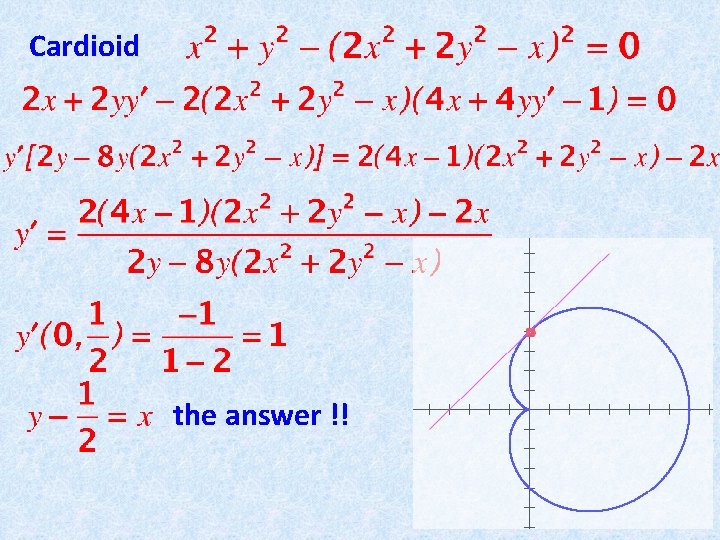# IMPLICIT DIFFERENTIATION AND RELATED RATES Once again we

• Slides: 9IMPLICIT DIFFERENTIATION AND RELATED RATES Once again we are going to look at two separate and apparently distinct situations that, not surprisingly, are resolved with the same mathematical model. Situation no. 1: Some very nice looking and useful curves in the plane are NOT describable as (i. e. , graphs of functions) but rather as i. e. solution sets of equations. Compute equations of tangent lines.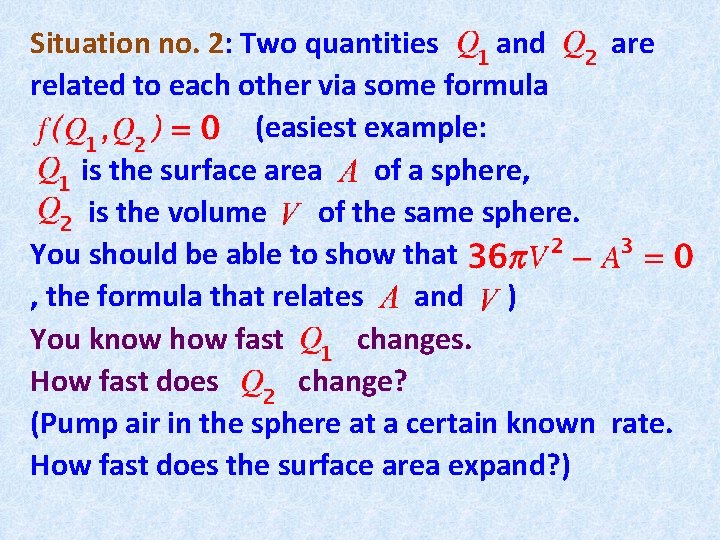Situation no. 2: Two quantities and are related to each other via some formula (easiest example: is the surface area of a sphere, is the volume of the same sphere. You should be able to show that , the formula that relates and ) You know how fast changes. How fast does change? (Pump air in the sphere at a certain known rate. How fast does the surface area expand? )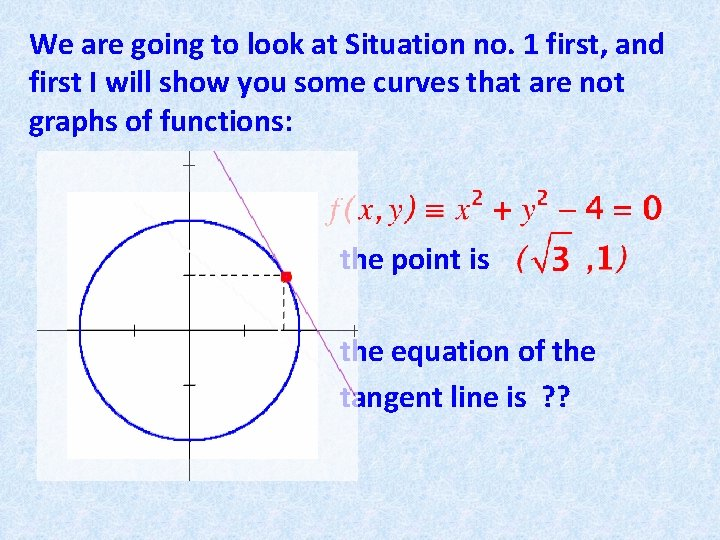We are going to look at Situation no. 1 first, and first I will show you some curves that are not graphs of functions: the point is the equation of the tangent line is ? ?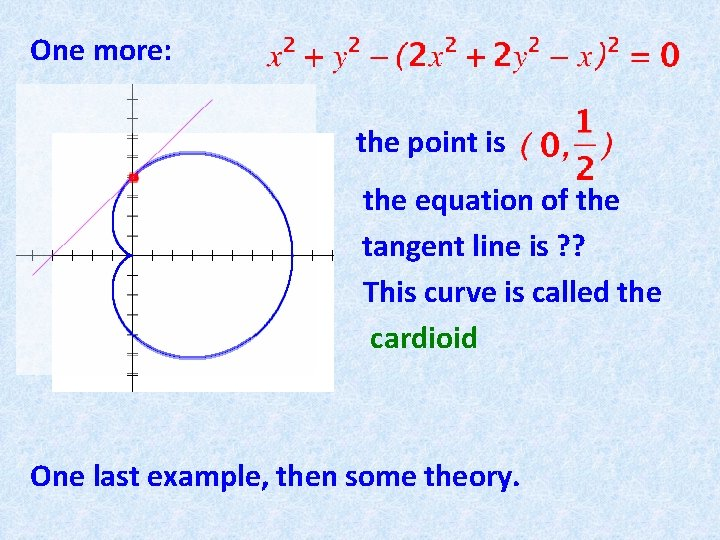One more: the point is the equation of the tangent line is ? ? This curve is called the cardioid One last example, then some theory.Last example: the point is (check that it’s on !) the equation of the tangent line is ? ? This curve is called the lemniscate Now to theory. Actually it is rather simple. Look at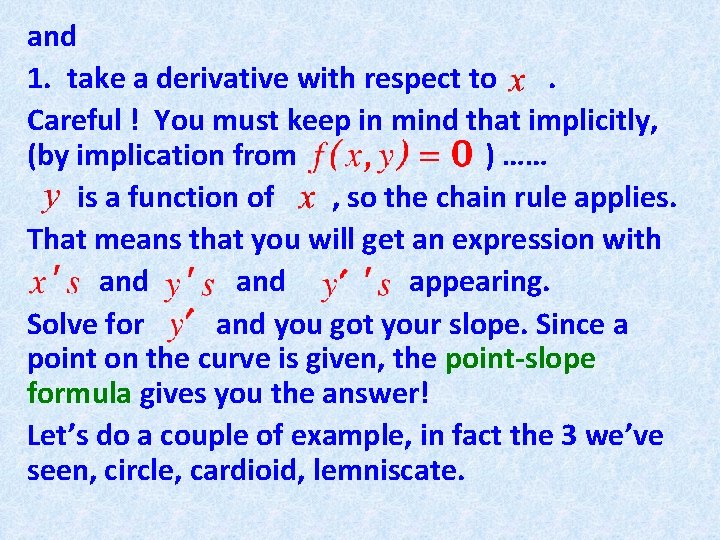and 1. take a derivative with respect to. Careful ! You must keep in mind that implicitly, (by implication from ) …… is a function of , so the chain rule applies. That means that you will get an expression with and appearing. Solve for and you got your slope. Since a point on the curve is given, the point-slope formula gives you the answer! Let’s do a couple of example, in fact the 3 we’ve seen, circle, cardioid, lemniscate.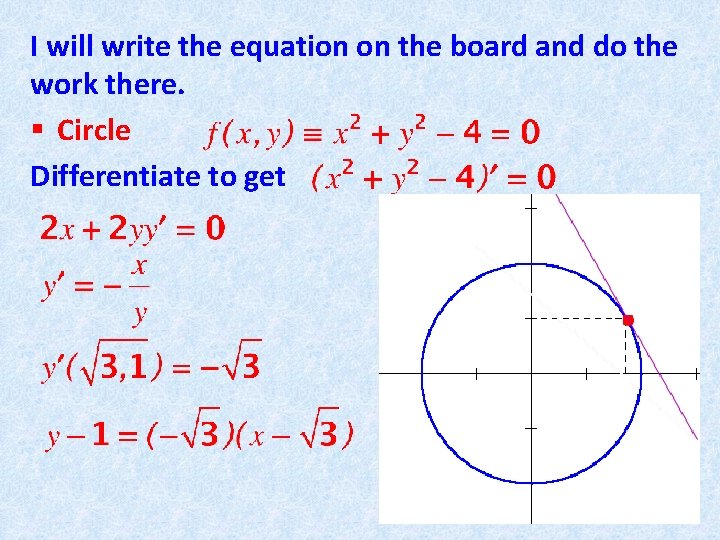I will write the equation on the board and do the work there. § Circle Differentiate to get